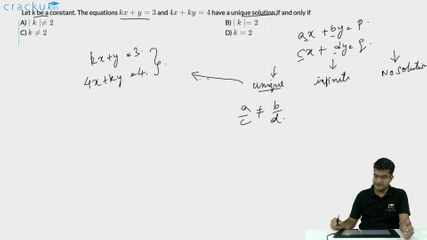Question 68

# Let k be a constant. The equations $$kx + y = 3$$ and $$4x + ky = 4$$ have a unique solution if and only if

Solution

Two linear equations ax+by= c and dx+ ey = f have a unique solution if $$\frac{a}{d}\ne\ \frac{b}{e}$$

Therefore, $$\frac{k}{4}\ne\ \frac{1}{k}$$ => $$k^2\ne\ 4$$

=> k $$\ne\$$ |2|

### View Video Solution